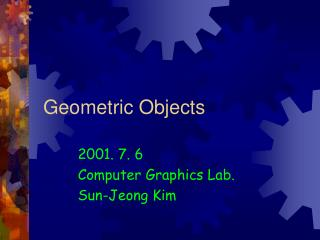DownloadDownload PresentationGeometric Objects

# Geometric Objects

Download Presentation## Geometric Objects

- - - - - - - - - - - - - - - - - - - - - - - - - - - E N D - - - - - - - - - - - - - - - - - - - - - - - - - - -
##### Presentation Transcript

1. Geometric Objects 2001. 7. 6 Computer Graphics Lab. Sun-Jeong Kim

2. Points • Single Coordinate Position • Set the bit value(color code) corresponding to a specified screen position within the frame buffer y setPixel (x, y) x

3. Lines • Intermediate Positions between Two Endpoints • DDA, Bresenham’s line algorithms Jaggies = Aliasing

4. y2 y2 y2 y2 y1 y1 y1 y1 x1 x1 x1 x1 x2 x2 x2 x2 DDA Algorithm • Digital Differential Analyzer • Slope >= 1 • Unit x interval = 1 • 0 < Slope < 1 • Unit y interval = 1 • Slope <= -1 • Unit x interval = -1 • -1 < Slope < 0 • Unit y interval = -1

5. d > 0 : choose NE  : dnew= dold+a • d <= 0 : choose E  : dnew= dold+a+b Bresenham’s Line Algorithm • Midpoint Line Algorithm • Decision variable NE Q M P(xp, yp) E

6. Bresenham’s Algorithm(cont.) • Initial Value of d • Update d

7. Polygons • Filling Polygons • Scan-line fill algorithm • Inside-Outside tests • Boundary fill algorithm

8. Scan-Line Polygon Fill • Topological Difference between 2 Scan lines • y : intersection edges are opposite sides • y’ : intersection edges are same side y y’

9. Scan-Line Polygon Fill (cont.) • Edge Sorted Table B yC yB xC 1/mCB C C’ E yD yC’ xD 1/mDC yE xD 1/mDE D yA yE xA 1/mAE yB xA 1/mAB A 1 Scan-Line Number 0

10. Inside-Outside Tests • Self-Intersections • Odd-Even rule • Nonzero winding number rule exterior interior

11. Boundary-Fill Algorithm • Proceed to Neighboring Pixels • 4-Connected • 8-Connected

12. Antialiasing • Aliasing • Undersampling: Low-frequency sampling • Nyquist sampling frequency: • Nyquist sampling interval:

13. Antialiasing (cont.) • Supersampling (Postfiltering) • Pixel-weighting masks • Area Sampling (Prefiltering) • Pixel Phasing • Shift the display location of pixel areas • Micropositioning the electron beam in relation to object geometry

14. Supersampling • Subpixels • Increase resolution 22 (10, 20): Maximum Intensity (11, 21): Next Highest Intensity (11, 20): Lowest Intensity 21 20 10 11 12

15. Pixel-Weighting Masks • Give More Weight to Supixels Near the Center of a Pixel Area 1 2 1 2 4 4 1 2 1

16. Area Sampling • Set Each Pixel Intensity Proportional to the Area of Overlap of Pixel • 2 Adjacent vertical (or horizontal) screen grid lines  trapezoid 22 (10, 20): 90% (10, 21): 15% 21 20 10 11 12

17. Filtering Techniques • Filter Functions (Weighting Surface) Box Filter Cone Filter Gaussian Filter

18. Mathematics for CG

19. Coordinate Reference Frames • 2D Cartesian Reference Frames x y y x

20. 2D Polar Coordinate Reference Frame

21. 3D Cartesian Reference Frame • Right-Handed v.s. Left-Handed Right-handed Left-handed

22. 3D Curvilinear Coordinate Systems • General Curvilinear Reference Frame • Orthogonal coordinate system • Each coordinate surfaces intersects at right angles

23. Cylindrical-Coordinate z axis constant : radius of vertical cylinder : vertical plane containing z-axis : horizontal plane parallel to xy-plane y axis Transform to Cartesian coordinator x axis

24. Spherical-Coordinate z axis constant : radius of sphere : vertical plane containing z-axis : cone with the apex at the origin y axis Transform to Cartesian coordinator x axis

25. Solid Angle • 3D Angle Defined on a Sphere • Steradian Steradian : Total solid angle : steradian

26. Points & Vectors • Point • Position in some reference frame • Distance from the origin depends on the reference frame P y Frame B Frame A x

27. Points & Vectors (cont.) • Vector • Difference between two point positions • Properties : Magnitude & direction • Same properties within a single coordinate system • Magnitude is independent from coordinate frames Magnitude : Direction :

28. 3D Vector • Magnitude • Directional angle

29. Vector Addition &Scalar Multiplication • Addition • Scalar multiplication

30. Vector Multiplication • Scalar Product(Inner Product) Commutative : Distributive : Orthogonal :

31. Vector Multiplication (cont.) • Vector Product(Cross Product) Noncommutative : Nonassociative : Distributive : Right-handed rule!